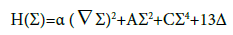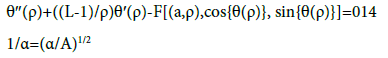###### Indexed In
• Open J Gate
• Genamics JournalSeek
• Smithers Rapra
• RefSeek
• Directory of Research Journal Indexing (DRJI)
• Hamdard University
• EBSCO A-Z
• OCLC- WorldCat
• Scholarsteer
• Publons
• Geneva Foundation for Medical Education and Research

### 9th International Conference on Advances in Chemical Engineering & Technology

Bangkok, Thailand

### 4th International Conference on Oil, Gas and Petro Chemical Sciences

Amsterdam, Netherlands

### 7th International Conference on Chemical Engineering & Medicinal Chemistry

Barcelona, Spain
###### Journal Flyer###### Open Access Journals

Research Article - (2017) Volume 7, Issue 1

## Comparison of Classical Nucleation Theory and Modern Theory of Phase Transition

Baranov SA1,2,3*
1Institute of Applied Physics, Academy of Sciences of Moldova, Republic of Moldova
2Department de Genie Physique, Ecole Polytechnique de Montreal, Montreal H3C 3A7, Quebec, Canada
3Shevchenko Transnistria State University, Tiraspol, Republic of Moldova
*Corresponding Author: Baranov SA, Department de Genie Physique, Ecole Polytechnique de Montreal, Montreal H3C 3A7, Quebec, Canada, Tel: +37322731725 Email:

## Abstract

The formation of electrochemical phase is a typical example of a first-order phase transition. An overview on old and new thermodynamic and kinetic aspects of the theoretical description of first-order phase transitions, and, in particular, of the theory of nucleation is given. Electrochemical nucleation of nanostructures has been considered in terms of the classical Gibbs and the Cahn-Hilliard-Hillert theories. We obtained agreement between the theories Kahn-Hilliard-Hillert and Gibbs.

Keywords: Electrochemistry; Nucleation; Nanoparticles; Phase transitions; Gibbs theory; Cahn-Hilliard theory

#### Introduction

Electrochemical nucleation is usually described in the framework of the classical Gibbs theory of nucleation, which provides a simple expression for the critical radius of a growing nucleus (nanoparticles) [1-11]:

rc=К(γ/μ) (1)

where γ is the specific surface energy, μ is the change in volume energy during a phase transition (e.g., nucleation), and K is the particle shape factor.

J ~ exp {-ΔEс/ kBT} (2)

where kB is the Boltzmann constant, and T is the absolute temperature.

The dimensionless parameter G, which is also referred to as the Gibbs number, describes, equilibrium electrochemical nucleation and has the form :

G=Δ Eс/kT (3)

Further, we briefly describe essential points of calculate ΔEc adopted in the classical Gibbs model first and then address modern theory of phase transitions.

#### Classical Gibbs Nucleation Theory

Energy ΔEс in the classical Gibbs nucleation theory (for a threedimensional case) was obtained by assuming that the nucleus is a sphere of radius r [1-9]:

where γ3 is the specific surface energy (for the three-dimensional case), μ3 is the change in volume energy during a phase transition (for the three-dimensional case).

The size of the critical radius of nucleation rc is determined in Equation 1 (where for the three-dimensional case: K3=2):

rc=2γ/μ

Critical nucleus radius corresponding to maximum G3 is as follows (Figure 1) [1-9]:

Figure 1: Qualitative kind diagram of the free energy barrier, ΔG3. The crossover of the bulk -1 and surface-2 terms combined with their opposing signs lead to the formation of a free energy barrier.

For a two-dimensional case, we obtain [1-9]where γ2 is the specific surface energy (for the two-dimensional case), μ3 is the change in volume energy during a phase transition (for the two-dimensional case).

The size of the critical radius of nucleation rc is determined in Equation 1 (where for the two-dimensional case: K2=1):

r2=γ/μ

Critical nucleus radius corresponding to maximum G2 is as follows [1-9]The generalization can be obtained for a multidimensional system:

And

ΔGn=Gnp/n (8)

According to Equation 7, in one-dimensional case,

r1c=0

because K1=0.

This finding is consistent with the general theory. In terms of the droplet model, for convex surfaces, one can obtain the following expression:

To calculate energy barrier ΔG, it is necessary to know the surface energy and particle shape factor k (i):

Energy barrier ΔG can be assumed to be proportional to cathode overpotential ηk (see, e.g., [5,10,11]). This dependence can be presented as follows:

It was experimentally studied for the case of n=2 and 3, which follows from Equations 8-12 (see, e.g., ). Other general findings are given below. Here, we note that, if the short-range interaction covers the following focal areas, then radius rnc increases to infinity, while the energy barrier vanishes. It was found that, for spinodal disintegration, the radius (which is similar radius rnc) increases indefinitely, while the energy barrier decreases.

#### Ginsburg-Landau-Abrikosov Theory And Cahn- Hilliard Theory Applied To Nucleation

The Ginsburg–Landau–Abrikosov theory uses free energy (Hamiltonian) in form [12-15]where order parameter Σ is a generalized spinor (linear term is omitted).

Function H(Σ) extreme condition allows deriving a differential equation. This equation was obtained in [14,15]:where L determines the space dimensionality of spinor (nDdimensionality), and F[(a,ρ),cos{θ(ρ)}, sin{θ(ρ)}] is a polynomial (analytical function) that can be defined in each case.

Application of Equation 14 to a 2D problem was thoroughly considered in . Below, we consider a 1D case (L=1), which corresponds to the Cahn-Hilliard-Hillert theory [16-22].

The resulting equation has the form

θ″(ρ)=(a/2)sin{2θ(ρ)} (15)

which is similar to previously reported equations [16-22] and according to which it is possible to obtain the solution :

cos{θ(ρ)}=-th{aρ)}(16)

The size of the domain wall is an important parameter determining the nucleating seed size (Figure 2):

Figure 2: Schematic representation of the solution [16,17] in the form of a domain wall of energy vectors (generalized spinor).

Assuming that the domain wall size determines the critical radius of a nanoparticle, the difference between Equations 1 and 17 should be noted.

It can be shown that the energy of the domain wall is also different from the classical formulas given by Equations 5 and 8:

Agreement between the Ginsburg-Landau-Abrikosov (Cahn- Hilliard-Hillert) theories and the classical Gibbs nucleation theory is discussed below.

#### Unification of the Theories

The simplest assumption regarding the relationship between the surface tension and the nanoparticle radius can be expressed as follows:

where Δin is the thickness of the surface layer of the nanoparticle and1 is a parameter of length characterizing the nanoparticle size in terms of Δin .

If rrel<1, then the Gibbs theory is not applicable. However, it can be applied in accordance with the Cahn-Hilliard-Hillert theory. In this case, a formula similar to Equation 1 (or Equation 7 without taking into account the kn factor) can be written as follows:

It is not difficult to transit to the formulas in the form of Equation 17 from the Cahn–Hilliard–Hillert theory:

If

Thus, there is a correspondence between the Cahn-Hilliard-Hillert and Gibbs theories. It is of interest to show that there is no contradiction between the formulas for the activation energy in the Cahn-Hilliard- Hillert and Gibbs theories.

In fact, the order of accuracy for activation energy is determined by the energy (barrier) necessary for merging particles, which is a product of the particle chemical potential (-μ) and the defect size (linear case implied) and can be estimated via Equation 21.

Finally, it is not difficult to arrive at the following formula:

Therefore, there is no contradiction between the Cahn-Hilliard- Hillert and Gibbs theories.

#### Conclusions

The formation of electrochemical phase is a typical example of a first-order phase transition. Electrodeposition of nanostructures has been considered in terms of the classical Gibbs and the Cahn-Hilliard- Hillert theories. Agreement between the theories Kahn-Hilliard- Hillert and Gibbs was obtained. We found no contradiction between the theories Cahn-Hilliard-Hillert and Gibbs. We have reviewed the progress of the modern thermodynamic theory of the growth of nanoparticles. We note the existence and of other approaches of the theory, presented for example in [23-26].

#### References

1. Gibbs JW (1928) Graphical methods in the thermodynamics of fluids. Yale University Press.
2. Frenkel J (1939) Statistical Theory of Condensation Phenomena. J Chem Phys 7: 200
3. Gamburg Y, Zangari G (2011) Theory and Practice of Metal Electrodeposition. Springer, New York, USA, p: 375
4. Wagner CZ (1961) Theorie der Alterung von Niderschlagen durch Umlösen (Ostwald Reifung). Electrochem. 65: 581-591.
5. Anisimov MA (1991) Critical Phenomena in Liquids and Crystals. Philadelphia, Gordon & Breach, USA, p:  280
6. Abraham FF (1974) Homogeneous Nucleation Theory. Academic Press, New York and London
7. Vetter KJ (1967) Electrochemical Kinetics: Theoretical Aspects. Academic Press, New York, USA, p: 726
8. Landau LD (1937) On the Theory of Phase Transitions 1. Phys Zs Sowjet 11: 26.
9. Landau LD (1937) On the Theory of Phase Transitions 2. Phys Zs Sowjet 11: 545.
10. Baranov SA (2015) Handbook of Nanoelectrochemistry: Electrochemical Synthesis Methods, Properties and Characterization Techniques. Springer, Switzerland, pp: 1057-1069
11. Cahn JW, Hilliard JE (1958) Free Energy of a Nonuniform System. I. Interfacial Free Energy. J Chem Phys. 28: 258
12. Cahn JW (1959) Free Energy of a Nonuniform System. II. Thermodynamic Basis. J Chem Phys 30: 1121
13. Cahn JW, Hilliard JE (1959) Free Energy of a Nonuniform System. III. Nucleation in a Two-Component Incompressible Fluid. J Chem Phys 30: 688
14. Cahn JW, Larche FC (1982) Surface stress and the chemical equilibrium of small crystals-II. Solid particles embedded in a solid matrix. Acta Met 30: 51
15. Hillert M (1975) Diffusion and interface control of reactions in alloys. Metall Trans. 6: 5
16. Khachaturyan AG (1983) Theory of Structural Transformations in Solids. Wiley, New York
17. Schmelzer JWP (2002) In: Encyclopedia of Surface and Colloid Science. Marcel Dekker Inc., New York
18. Gutzow I, Schmelzer J (1995) The Vitreous State: Thermodynamics, Structure, Rheology, and Crystallization. Springer, Berlin, Germany
19. Schmelzer JWP, Roepke G, Priezzhev VB (1999) Nucleation Theory and Applications. Joint Institute for Nuclear Research Publishing House, Dubna, Russia.
20. Schmelzer JWP, Roepke G, Priezzhev VB (2002) Nucleation Theory and Applications. Joint Institute for Nuclear Research Publishing House, Dubna, Russia.
Citation: Baranov SA (2017) Comparison of Classical Nucleation Theory and Modern Theory of Phase Transition. J Adv Chem Eng 7: 177.

Copyright: Â© 2017 Baranov SA. This is an open-access article distributed under the terms of the Creative Commons Attribution License, which permits unrestricted use, distribution, and reproduction in any medium, provided the original author and source are credited.